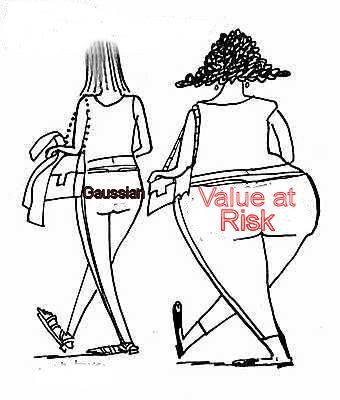Apr 9

## Value at Risk Estimation with Copula

Posted by abiao at 09:29 | Code » Other | Comments(8) | Reads(22723)
Value at Risk is widely used to measure the downside risk, and Copula is a generalized dependence structure instead of linear correlation to model dependence, especially for lower tail dependence, therefore the combination of VaR with Copula is fantastic in terms of accurately capturing the true risk embedded.I read roughly a working paper Value at Risk – MATLAB Application of Copulas on US and Indian Markets, where the authors calculate the Value at Risk (VaR) using the bivariate Gaussian Copula distribution implemented in MATLAB for the Dow-Jones index and the National Stock Exchange index. It is good to use Gaussian Copula together with some rank correlation (like Spearman's rho or Kendall tau) to model the dependence, however, we must be very careful as basically Gaussian Copula assumes the joint dependence structure normally distributed and as a result, no matter which marginal distribution you choose, the upper and lower tail dependence approach to zero when the significant level limits to one and zero, in other words, in a bivariate case, the probability that X2 exceeds its q-quantile, given that X1 exceeds its q-quantile (upper tail dependence) when q->1, and the probability that X2 is below its q-quantile, given that X1 is below its q-quantile (lower tail dependence) when q->0 are zero.

For example, below are two simulated return series, one is under Gaussian copula and the other one is under Student t copula, as you can easily see, although both have the same marginal distribution, Gaussian copula has much smaller upper and lower tail dependence than Student t copula, which eventually underestimates the Value at Risk and other risk measures.I would stay away Gaussian Copula if I were a risk manager, and you? Download Copula toolbox and other code files at Copula if interested.

Tags: ,
I would agree with you, as VaR is proved "inefficient" as a risk measure, does it really matter in which form we calculate it?
Well, whether VaR is efficient or not is another issue, we can't just use a less accurate method to calculate VaR because it is inefficient. Plus, other more efficient risk measure, like expected shortfall, still requires tail dependence modelling.
Tails are not only fat, but also asymmetrical that lower tail is fatter than upper one.  Theories always work well under their assumptions, but to what extent their assumptions are right?  It is hard to tell.  It would better to invent some tests to tell which distribution we should use, Gaussian, Student t, Archimedean or something else?
yeah, exactly, student t also has symmetric tail dependence, depending on the portfolio risk profile, some Archimedian copulas may be used.
I think that parametric models (also t-havy tails models) are unrealistic in the VaR implementation. Bayesian approach seems to be better in this way, because you can modelize the correlation with mixtures (even infinite mixture) of distributions and this allows better and realistic estimation in the tails.
Why is t model unrealistic? it has analytic solution and is easy to implement. I know little about Bayesian approach, my first impression is it may be less intuitive.
Yes i found an article that has very nice information. download game mobile legend
One of the greatest sites I've seen in a while.

nba live mobile hack
 Add a comment Emots Enable HTML Enable UBB Enable Emots Hidden Remember Nickname   Password   Optional Site URI   Email   [Register]